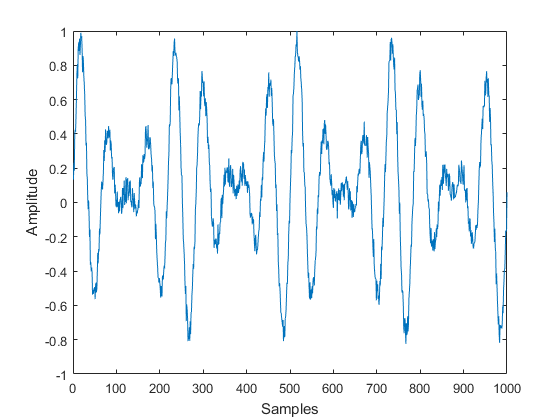This example shows how to use the Quick-Control Function Generator to generate arbitrary waveforms.

Instrument Control Toolbox™ supports communication with instruments through interfaces and drivers. For a complete list of supported hardware, visit the Instrument Control Toolbox product page at www.mathworks.com/products/instrument.html

### Introduction

In this example we will create and download an arbitrary waveform to an arbitrary waveform generator using Quick-Control Function Generator.

### Requirements

To run this example you need:

• An arbitrary waveform generator (This example uses Tektronix® AFG3022B).

• VISA software installed on your machine (This example uses Agilent® IO Libraries Version 16.1).

• IVI-C drivers for the instruments installed on your machine (This example uses Tektronix® tkafg3k IVI-C driver version 3.2 from the IVI Foundation Driver Registry).

• Instrument Control Toolbox™.

### Define waveform parameters

We will create an arbitrary waveform that consists of three different waveforms. Each waveform's properties, including the amplitude and frequency,will be set in the section below. For each waveform, the amplitude is in `volts`, while the frequency is in `Hz`.

When generating signals for a function generator it is important to ensure continuity in the time domain so as to not introduce unintended spectral content in the signal, especially if the waveform is going to be played back repeatedly. To ensure continuity you can define the time vector such that it contains an integral number of cycles of each of the three tones that will compose the synthesized waveform.

```timeStep = 0.001; time = 0:timeStep:(1-timeStep); ```

Parameters for the first waveform

```amplitude1 = 0.2; frequency1 = 10; ```

Parameters for the second waveform

```amplitude2 = 0.8; frequency2 = 14; ```

Parameters for the third waveform

```amplitude3 = 0.6; frequency3 = 18; ```

### Create arbitrary waveform

We will create our three individual waveforms using the `sin` command.

```waveform1 = amplitude1*sin(2*pi*frequency1*time); waveform2 = amplitude2*sin(2*pi*frequency2*time); waveform3 = amplitude3*sin(2*pi*frequency3*time); ```

The arbitrary waveform will be a combination of each of the above listed waveforms.

```waveform = waveform1 + waveform2 + waveform3; ```

Add random noise to the waveform created earlier.

```waveform = waveform + 0.3*rand(1,size(waveform,2)); ```

Some function generators require a normalized waveform. In this case the waveform is normalized between -1 to +1.

```waveformArray = (waveform./max(waveform))'; plot(waveformArray); xlabel('Samples'); ylabel('Amplitude'); ```Discover all the available instrument resources (targets) you can connect to, using the `resources` command.

```f = fgen; f.resources ```
```ans = 1×211 char array ASRL1::INSTR ASRL3::INSTR ASRL::COM1 ASRL::COM3 GPIB0::INTFC PXI0::MEMACC TCPIP0::172.28.22.217::inst0::INSTR TCPIP0::172.28.23.55::inst0::INSTR TCPIP0::a-d6054l-000006.dhcp.mathworks.com::inst0::INSTR ```

### Connect to function generator

Now that you have your waveform you need to download it onto the function generator. You will use the Quick-Control Function Generator or `fgen` function of the Instrument Control Toolbox™ to do so. Because the IP address of the instrument is 172.28.22.217, the resource specified will be TCPIP0::172.28.22.217::inst0::INSTR.

```f = fgen('TCPIP0::172.28.22.217::inst0::INSTR','tkafg3k'); ```

Specify the channel of the function generator where the waveform would be generated. Here, the waveform would be generated on channel number 1.

```selectChannel(f, '1'); ```

Since you will be generating a custom arbitrary waveform, set the `Waveform` property to Arb.

```f.Waveform = 'Arb'; ```

```downloadWaveform (f, waveformArray); ```

Enable the waveform generation.

```enableOutput(f); ```

### Clean up

```clear f; ```

## SupportGet trial now